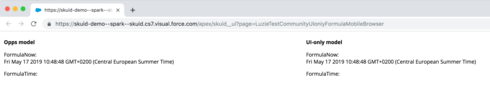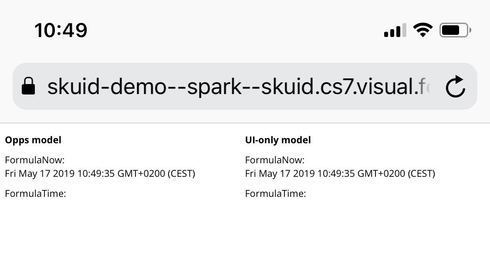# UI Only formula fields not working on mobile browsers?

edited October 2, 2019
Hi team,

I've come across an issue where some UI only formula fields don't display in mobile browsers in IOS (tested both Chrome and Safari). Chrome on Android behaves like the desktop counterparts.

I'm using a formula to output text using this syntax (but other output types seem to be affected as well with different formulas):

FORMAT_TIME("hh:mma",YEAR(TODAY())+"-"+MONTH(TODAY())+"-"+DAY(TODAY())+" "+LEFT({{TimeEndMorning__c}},5)+":00")

Why wouldn't these UI formula fields work on IOS and how can I make sure they do? I would very much prefer a solution using UI formulae as opposed to doing this in Salesforce.

The org is on Spark 12.0.8.

Robin

• Would love to know if this is a known issue :-) Much appreciated!
• It would be amazing to get an official response since this is creating major headaches and we're losing money with no workaround other than creating all fields in Salesforce, which isn't viable in that org. Many thanks
• If we could please get an official response or at least an acknowledgement for this, that would be great. Thanks in advance
• Hi Robin, so far I couldn't reproduce the issue that you described. I tested a simple formula and your formula, too. On my test page, I can see the simple formula in a desktop browser (Chrome) as well as in a mobile browser on iOS (Safari). However, your formula didn't show a result in either one. Could you please check the following test page and let me know your result?

This is what I see in a desktop browser (Chrome):This is what I see in a mobile browser on iOS (Safari):Skuid page XML:

<models>
<model id="UIonly" limit="20" query="true" createrowifnonefound="true" datasource="Ui-Only" processonclient="true">
<fields>
<field id="FormulaNow" displaytype="FORMULA" label="FormulaNow" ogdisplaytype="TEXT" readonly="true" returntype="TEXT" defaultvaluetype="fieldvalue">
<formula>NOW()</formula>
</field>
<field id="FormulaTime" displaytype="FORMULA" label="FormulaTime" ogdisplaytype="TEXT" readonly="true" returntype="TEXT">
<formula>FORMAT_TIME("hh:mma",YEAR(TODAY())+"-"+MONTH(TODAY())+"-"+DAY(TODAY())+" "+LEFT({{TimeEndMorning__c}},5)+":00")</formula>
</field>
</fields>
<conditions/>
<actions>
<action>
<actions>
<action type="save">
<models>
<model>UIonly</model>
</models>
</action>
</actions>
<events>
</events>
</action>
</actions>
</model>
<model id="Opps" limit="1" query="true" createrowifnonefound="false" datasource="salesforce" sobject="Opportunity">
<fields>
<field id="FormulaNow" uionly="true" displaytype="FORMULA" label="FormulaNow" defaultvaluetype="fieldvalue" ogdisplaytype="TEXT" readonly="true" returntype="TEXT">
<formula>NOW()</formula>
</field>
<field id="FormulaTime" uionly="true" displaytype="FORMULA" label="FormulaTime" ogdisplaytype="TEXT" readonly="true" returntype="TEXT">
<formula>FORMAT_TIME("hh:mma",YEAR(TODAY())+"-"+MONTH(TODAY())+"-"+DAY(TODAY())+" "+LEFT({{TimeEndMorning__c}},5)+":00")</formula>
</field>
</fields>
<conditions/>
<actions/>
</model>
</models>
<components>
<grid uniqueid="sk-34yI-1659">
<divisions>
<division behavior="flex" minwidth="100px" ratio="1">
<components>
<richtext multiple="false" uniqueid="sk-34uo-412" model="Opps">
<contents>&lt;p&gt;&lt;strong&gt;Opps model&lt;/strong&gt;&lt;/p&gt;

&lt;p&gt;FormulaNow:&lt;br /&gt;
{{FormulaNow}}&lt;/p&gt;

&lt;p&gt;FormulaTime:&lt;br /&gt;
{{FormulaTime}}&lt;/p&gt;
</contents>
</richtext>
</components>
</division>
<division behavior="flex" verticalalign="top" minwidth="100px" ratio="1">
<components>
<richtext multiple="false" uniqueid="sk-34u2-229" model="UIonly">
<contents>&lt;p&gt;&lt;strong&gt;UI-only model&lt;/strong&gt;&lt;/p&gt;

&lt;p&gt;FormulaNow:&lt;br /&gt;
{{FormulaNow}}&lt;/p&gt;

&lt;p&gt;FormulaTime:&lt;br /&gt;
{{FormulaTime}}&lt;/p&gt;
</contents>
</richtext>
</components>
</division>
</divisions>
<styles>
<styleitem type="background" bgtype="none"/>
</styles>
</grid>
</components>
<resources>
<labels/>
<javascript/>
<css/>
<actionsequences uniqueid="sk-34to-100"/>
</resources>
<styles>
<styleitem type="background" bgtype="none"/>
</styles>
</skuidpage>

• I am also having the same issue. Is there any solution instead of this.
• edited October 2, 2019
1. Which Skuid version?
2. V1 or V2 page?
3. What happens if you create a new page with my repro XML above?
4. Please share screenshots of your results on a desktop computer and a mobile device.
5. Which browser is used on the desktop computer?
6. Which mobile device?
7. Which browser?